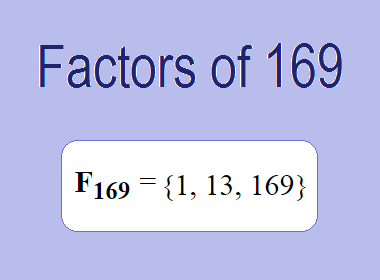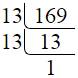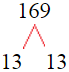# Factors of 169The factors of 169 are 1, 13, and 169 i.e. F169 = {1, 13, 169}. The factors of 169 are all the numbers that can divide 169 without leaving a remainder.

We can check if these numbers are factors of 169 by dividing 169 by each of them. If the result is a whole number, then the number is a factor of 169. Let's do this for each of the numbers listed above:

·        1 is a factor of 169 because 169 divided by 1 is 169.

·        13 is a factor of 169 because 169 divided by 13 is 13.

·        169 is a factor of 169 because 169 divided by 169 is 1.

## How to Find Factors of 169?

1 and the number itself are the factors of every number. So, 1 and 169 are two factors of 169. To find the other factors of 169, we can start by dividing 169 by the numbers between 1 and 169. If we divide 169 by 2, we get a remainder of 1. Therefore, 2 is not a factor of 169. If we divide 169 by 3, we get a remainder of 1. Therefore, 3 is not a factor of 169.

Next, we can check if 4 is a factor of 169. If we divide 169 by 4, we get a remainder of 1. Therefore, 4 is not a factor of 169. We can continue this process for all the possible factors of 169.

Through this process, we can find that the factors of 169 are 1, 13, and 169. These are the only numbers that can divide 169 without leaving a remainder.

********************

********************

## Properties of the Factors of 169

The factors of 169 have some interesting properties. One of the properties is that the sum of the factors of 169 is equal to 183. We can see this by adding all the factors of 169 together:

1 + 13 + 169 = 183

Another property of the factors of 169 is that they are all odd numbers. This is because 169 is an odd number, and any even number cannot divide an odd number without leaving a remainder.

Another property of the factors of 169 is that the prime factor of 169 is 13 only.

## Applications of the Factors of 169

The factors of 169 have several applications in mathematics. One of the applications is in finding the highest common factor (HCF) of two or more numbers. The HCF is the largest factor that two or more numbers have in common. For example, to find the HCF of 169 and 26, we need to find the factors of both numbers and identify the largest factor they have in common. The factors of 169 are 1, 13, and 169. The factors of 26 are 1, 2, 13, and 26. The largest factor that they have in common is 13. Therefore, the HCF of 169 and 26 is 13.

Another application of the factors of 169 is in prime factorization. Prime factorization is the process of expressing a number as the product of its prime factors. The only prime factor of 169 is 13. We can express 169 as:

169 = 13 × 13

We can do prime factorization by division and factor tree method also. Here is the prime factorization of 169 by division method,169 = 13 × 13

Here is the prime factorization of 169 by the factor tree method,169 = 13 × 13

## Conclusion

The factors of 169 are the numbers that can divide 169 without leaving a remainder. The factors of 169 are 1, 13, and 169. The factors of 169 have some interesting properties, such as being odd numbers and having a sum of 183. The factors of 169 have several applications in mathematics, such as finding the highest common factor and prime factorization.